• English
• Account
• API
• Integrations
• Enterprise
• Resources
Basics of numeric functions
• 04 Oct 2022
• Dark
Light

# Basics of numeric functions

• Dark
Light

Article Summary

Different functions work with different data types. In this article, we'll take a basic look at functions that work exclusively with numbers. These functions go beyond the calculations you can do with the four numeric operators to give you more ways to read, transform, and manipulate numeric values.

To introduce you to numeric functions, we'll cover the following functions. Hover over any of them to learn what they do and click them to jump to more detail.

• `MAX()`
• `MIN()`
• `AVERAGE()`
• `SUM()`

Of course, you can simply scroll through each section to learn the detail about the functions above as well.

## MAX() and MIN()

These two functions work similarly to return either the largest or smallest of given numbers. In the example below, you can easily find the largest of several payments by using this formula:

``MAX( {Payment 1} , {Payment 2} , {Payment 3} )``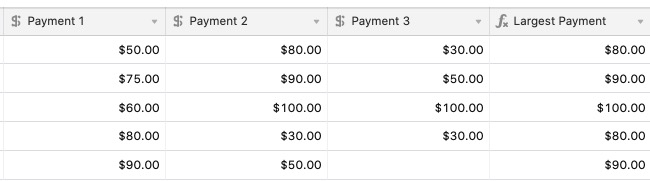You could alter this to `MIN({Payment 1},{Payment 2},{Payment 3})` to find the smallest payment.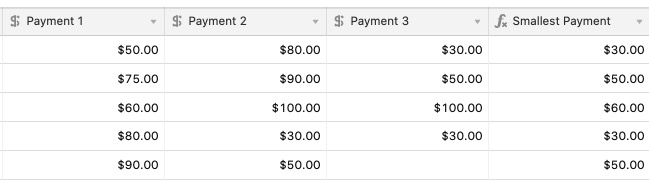Notice that only the fields with values entered are counted for this function. The empty value in {Payment 3} isn't counted as the lowest value since there is no actual value in that field.

## AVERAGE()

The AVERAGE() function returns the average of the numbers referenced within the function. For example, `AVERAGE(5, 10, 15)` results in `10`. Similar to `SUM()`, you can use this function to reference individual values or field names containing values.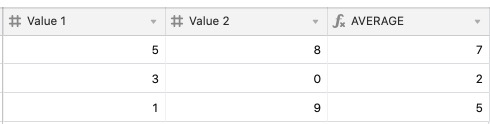``````AVERAGE ({Value 1 },{Value 2 })
``````

As another example, let's say you wanted to build a table of all the restaurants you're planning to visit on vacation. You plan to rate each one based on their food, service, and atmosphere, and you want to calculate the average score for each place.Ratings are stored as numeric values; each star is a value of 1 Using `AVERAGE()`, you can easily assign an average rating for each restaurant visited using the formula `AVERAGE({Food}, {Atmosphere}, {Service}).`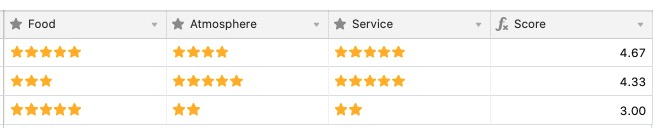## SUM()

The SUM() function allows you to find the sum, or total, of any amount of numeric values. The function is written as: `SUM(values)`.

This is equivalent to manually adding each value using the `+` operator, like `Number 1 + Number 2`. You can use this function to individually reference numbers or to reference a field name that contains numeric values.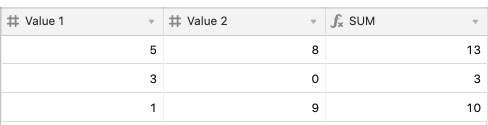``````SUM ({Value 1 }, {Value 2 }
``````

A common question is if it's possible to sum an entire field of numbers. Because formulas apply across fields (or in other words, to a specific record), and not vertically down fields (like you can in a traditional spreadsheet application), you cannot use a formula in a field to sum the amount of that field.

There are two alternatives however to finding the sum of a field:

1. Using the summary bar
2. Using linked records and a rollup field

## Using the summary bar

You can sum a range of cells by selecting a cell, holding SHIFT, and then clicking a different cell. The sum will show in the summary bar.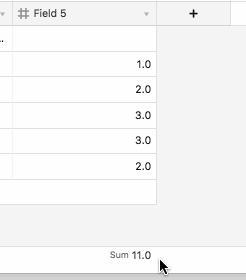## Using linked records and a rollup field

You can sum an entire field by taking the following steps:

1. Create an additional field, and populate every record in the field with the same value. You can do this quickly by using the fill-handle.
2. Convert the field to a linked record field that links to a new table.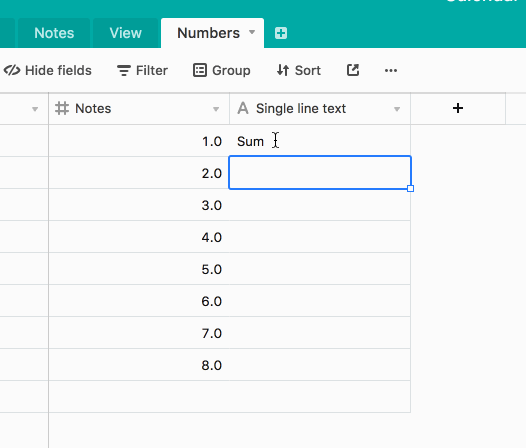3. On the new table, create a Rollup field that sums the number field with the Sum(values) function.